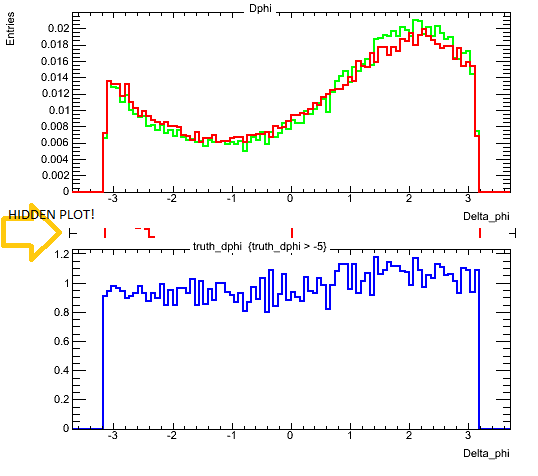# Hidden plot in TCanvas divide!

Hi everyone,

I’m having troubles in TCanvas divide using the function c1.Divide(1,2). In fact, I noticed that, beyond the two plots in c1.cd(1) and c1.cd(2), there is another plot! You can see it in the attached picture.

Thank you!

Here the code, written in PyROOT:

[code]from ROOT import *
import ROOT
import sys

intree_1 = infile_1.Get(“tree;1”)
intree_2 = infile_2.Get(“tree;1”)

intree_1.Draw(“truth_dphi >> h1”,“truth_dphi > -5”)
intree_1.Draw(“truth_dphi >> h4”,“truth_dphi > -5”)
intree_2.Draw(“truth_dphi >> h2”,“truth_dphi > -5”)

c1.Divide(1,2)

int1 = h1.Integral(“weight”)
int2 = h2.Integral(“weight”)
int4 = h4.Integral(“weight”)

h1.Scale(1./int1)
h2.Scale(1./int2)
h4.Scale(1./int4)

h1.SetTitle(“Dphi”)

c1.cd(1)
h1.GetXaxis().SetTitle(“Delta_phi”);
h1.GetYaxis().SetTitle(“Entries”)
h1.SetLineColor(kGreen)
h2.SetLineColor(kRed)

h1.Draw()
h2.Draw(“same”)

c1.cd(2)
h4.Divide(h2)
h4.GetXaxis().SetTitle(“Delta_phi”);
h4.SetLineColor(kBlue)
h4.Draw()

c1.Draw()
c1.SaveAs(“test.root”)
[/code]Try to add “c1.Clear()” directly before “c1.Divide(1,2)”.

ok, it works! Many thanks!!